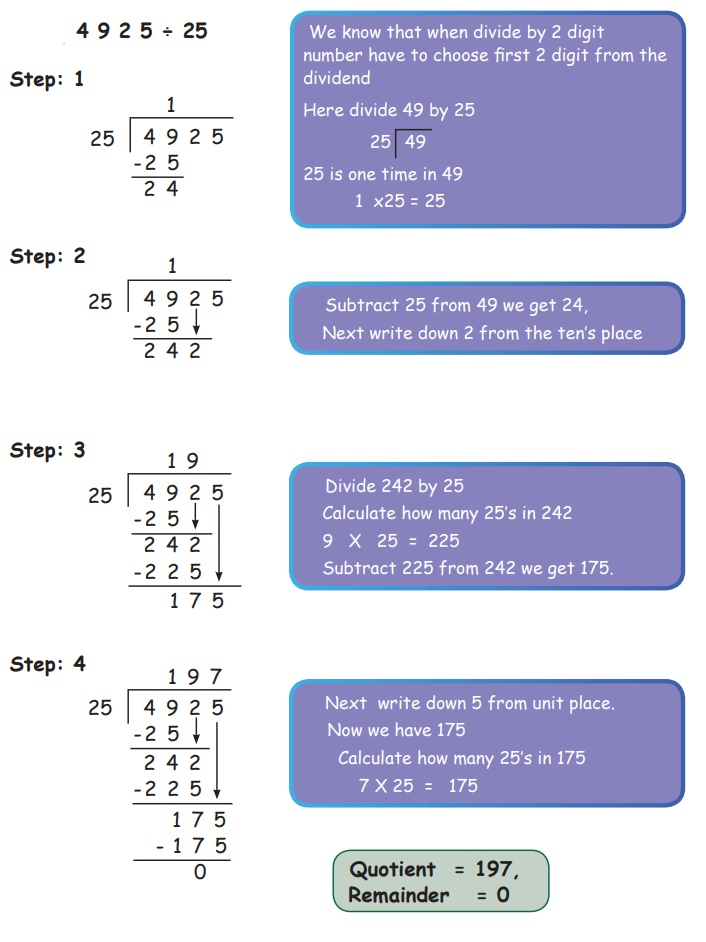Home | | Maths 5th Std | Divide 4 digits by 2 digits

# Divide 4 digits by 2 digits

We learnt how to divide a single digit in last class. Now we are going to learn how to divide a 4 digit number by a 2 digit number.

Divide 4 digits by 2 digits

We learnt how to divide a single digit in last class. Now we are going to learn how to divide a 4 digit number by a 2 digit number.On that day 5th std students are very excited, as the bus came to school for educational tour. The class teacher asked the students to get in the bus. Students got into the bus happily. The bus reached Arignar Anna Botanical garden. The class teacher paid ₹ 1530 as Entrance fee for all the students. If there are 34 students, what is the entrance fee for one student?

So, we have to divide the total amount ₹ 1530 by 34.

1530 ÷ 34Quotient = 45,

Remainder = 0

Therefore, the entrance fee for one student is ₹ 45

Divide and find the quotient and Remainder.

Example 1 : 4925 ÷ 25Quotient = 197

Remainder = 0’

Example 2: Divide 4327 by 18 and find quotient and remainder

Solution :Dividend = 4327

Divisor = 18

Quotient = 240

Remainder = 7

Example 3:

A car factory produces 3750 cars per month (30 days). Find the number of cars produced per day.

Divide 3750 by 30 days.

3750 ÷30Quotient = 125,

Remainder = 0

The remainder is zero. The remainder could not be zero for all problems.

Tags : Numbers | Term 1 Chapter 2 | 5th Maths , 5th Maths : Term 1 Unit 2 : Numbers
Study Material, Lecturing Notes, Assignment, Reference, Wiki description explanation, brief detail
5th Maths : Term 1 Unit 2 : Numbers : Divide 4 digits by 2 digits | Numbers | Term 1 Chapter 2 | 5th Maths